## What is Current Density?

By definition, the current density, denoted by the symbol J, is the current per unit area of the conducting medium i.e.,where

• current I is the rate of flow of charge.

If N is the free electron density moving in the conducting medium, e is the charge of electron, then the rate of flow of charge is given by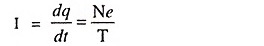Thus the current density J is given by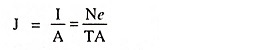But the time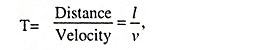Therefore,

Current density,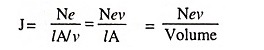where

• lA is the volume of conductor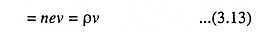where

• ρ = ne, the charge density, in coulombs per cubic metre and
• ν  = is the velocity in metres per second.

The above derivation is independent of the form of conducting medium. Consequently, it is not necessary that the conducting medium is a wire conductor. It may be equally well a portion of gaseous-discharge tube or a volume element in the space-charge cloud of a vacuum tube or semiconductor. Furthermore, neither ρ nor ν need be constant, but may vary from point to point in space or may vary with time.

Scroll to Top